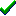# fg_polyfilz()Win32DirectXFastgraph/Light

Prototype

void fg_polyfilz (int *vertex_array, double *xyz_array, int n);

Sub fg_polyfilz (vertex_array() As Long, xyz_array() As Double, ByVal n As Long)

procedure fg_polyfilz (var vertex_array : integer; var xyz_array : byte; n : integer);

Description

The fg_polyfilz() function draws a projected z-buffered filled convex polygon in screen space, with 2D clipping. This function is called internally by Fastgraph's 3D functions and is not usually called directly by applications.

Parameters

vertex_array is the name of the array containing the (x,y) coordinate pairs of each polygon vertex. The first array element is the x component of the first vertex, the second element is the y component of the first vertex, the third element is the x component of the second vertex, and so forth.

xyz_array is the name of the array containing the 3D (x,y,z) coordinates for each (x,y) coordinate pair in vertex_array. The first three xyz_array elements represent the (x,y,z) values at the first vertex in vertex_array, the next three xyz_array elements are for the second vertex, and so forth. Only the z coordinates are meaningful in this function.

n is the number of vertices each of the above arrays.

Return value

none

Restrictions

If you attempt to fill a non-convex polygon with fg_polyfilz(), only a portion of the polygon will be filled.

See also

Contents
Fastgraph Home Page

copyright 2001 Ted Gruber Software, Inc.